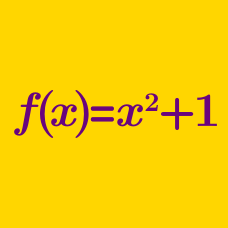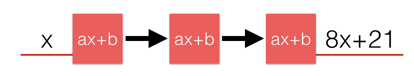Algebra

# Functions: Level 1 Challenges

If $a \oplus b = \left( a+b \right) ^{ 2 }$ , what is the value of $3\oplus 1$?

Consider a function $f$ satisfying $f(x) = | x^{3} - 7x |$. What is the value of $f(3)$?

If $f$ is a function such that $f(f(x)) = x^2 - 1$, what is the value of $f(f(f(f(3))))$?

Consider a function $f$ satisfying

$f\big(\sqrt{x}\big)=x^2.$

What is the value of $f(2) ?$If $f\left( x \right) =ax+b$, where $a$ and $b$ are real numbers, and $f\left( f\left( f\left( x \right) \right) \right) =8x+21$, what is $a+b$?

×

Problem Loading...

Note Loading...

Set Loading...